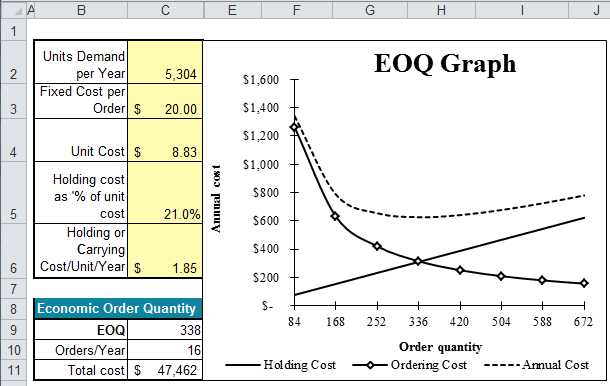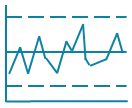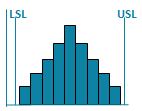# EOQ Calculator in Excel

## Calculate Economic Order Quantity (EOQ)

### To calculate EOQ you need to know:

1. Demand Per Year
2. Fixed Cost Per Order
3. Holding Cost Per Item (sometimes expressed as a percent of unit cost)

### EOQ Template for Excel

This economic order quantity calculator is just one of more than 140 templates in QI Macros add-in for Excel. QI Macros also draws spc charts like control charts, histograms and Pareto charts.

To calculate EOQ, simply input your data into the yellow shaded input areas. QI Macros does the rest.The Economic Order Quantity Calculator is just one of more than 140 templates in QI Macros add in for Excel.

### Other charts in QI Macros add-in

Control ChartsHistogramsPareto ChartsBox PlotsFishbone Diagram